# What is the Fatigue Damage Spectrum (FDS)?

December 11, 2020

In random vibration testing, the power spectral density (PSD) is the standard tool for analyzing a random waveform. The PSD introduces a test item to a vibration environment comparable to the real world over a set timeframe.

But what if we want to analyze a product’s total life damage?

A test engineer may want to know how a product will react to the accumulation of damage over many years. Fatigue testing is useful for predicting a product’s life expectancy and anticipating potential product failures with standard use.

### What is the Fatigue Damage Spectrum?

The fatigue damage spectrum (FDS) is a test development tool that replicates the operational environment of a product. It is used to create an accelerated random test using real-world data. The resulting test is the damage equivalent to a product’s lifespan.

The FDS is developed using recorded time-history files. From there, it can be used to create a test profile that replicates a lifetime of use (and damage).

### How the FDS is Calculated

The FDS computation is based on Miner’s rule of damage.1 According to this damage model, fatigue damage accumulates with exposure to stress cycles at different stress levels. The damage calculation is as follows (Equation 1):

(1)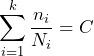Equation 1

Where:

• k is the stress levels
•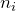is the number of stress cycles at ith stress level (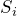)
•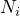is the average number of cycles to failure at• C is the fatigue/endurance limit

According to Miner’s rule, a product will fail when the fatigue limit (C) exceeds a value of 1.

### FDS Calculation Procedure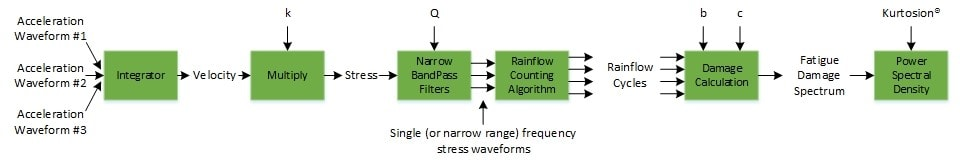To calculate the FDS, multiple time-history recordings are combined and weighted based on their contribution to the life of the product in a standard environment.

To start, a narrowband filter is applied to one or more recorded time-history waveforms. Each narrowband filter corresponds to a breakpoint in the PSD. Typically, these filters are spaced at an octave value (1/3 octave, 1/12 octave, etc.).

A rain flow algorithm counts the peak-to-valley stress cycles for each frequency band, and the stress cycle amplitudes are weighted non-linearly based on the power-law function of Miner’s rule. The combined stress cycles illustrate the fatigue of the product at each given frequency.

A data plot known as the S-N curve displays peak stress versus the total number of cycles to failure. The m value is calculated from the slope of the S-N curve (m = -1/b, where b = the slope of the S-N curve). Then, the m value and the resonance factor (Q) are used to calculate the fatigue damage value for each single/narrow range frequency waveform. The plots of the fatigue damage values make up the FDS.

For test purposes, the FDS can then be converted into a PSD using Henderson-Piersol’s potential damage spectrum.2 The result is a single PSD profile for multiple time-history files. The FDS-generated PSD is a cumulative spectrum that represents the relative damage experienced by the product for all the combined and weighted environments.

#### Non-Gaussian Waveforms

As the FDS is based on the rain flow analysis and cycle counting of time-domain waveforms, every peak to valley stress cycle is accounted for. Therefore, any non-Gaussian features of the time waveforms are also accounted for.

Other methods use a frequency domain conversion to move the time data into the frequency domain before performing the damage calculation. This process assumes the data are Gaussian and often results in an under test.

### What’s Next?

The FDS is used to create a vibration test profile that replicates a lifetime of product damage within the same time frame as the industry standard. First, however, the acceleration parameters must be determined. The m value, resonance factor (Q), target life, and test duration must be set by the test engineer before the FDS can be generated.